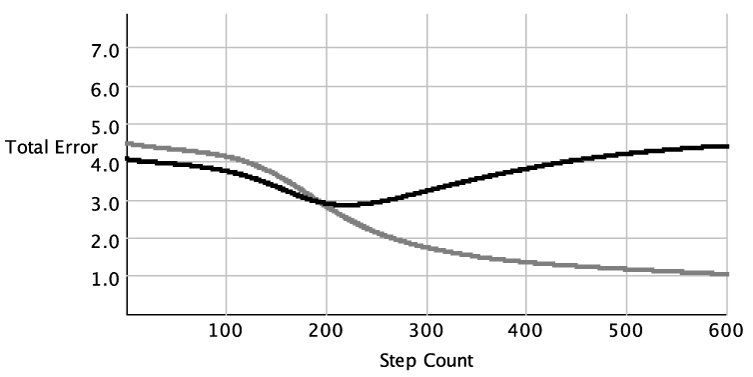## 7.5 Avoiding Overfitting

Overfitting can occur when some regularities appear in the training data that do not appear in the test data, and when the learner uses those regularities for prediction.Figure 7.13: Error as a function of training time. On the x-axis is the step count of a run of back-propagation with three hidden units on the data of Figure 7.9, using unseen examples as the test set. On the y-axis is the sum-of-squares error for the training set (gray line) and the test set (black line).

Example 7.17: Figure 7.13 shows a typical plot of how the sum-of-squares error changes with the number of iterations of linear regression. The sum-of-squares error on the training set decreases as the number of iterations increases. For the test set, the error reaches a minimum and then increases as the number of iterations increases. The same behavior occurs in decision-tree learning as a function of the number of splits.

We discuss two ways to avoid overfitting. The first is to have an explicit trade-off between model complexity and fitting the data. The second approach is to use some of the training data to detect overfitting.